Calculus Problem Solver 1.0

 Product Overview How to use Screen shots

 Get it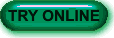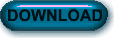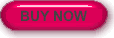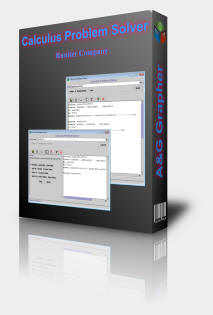Solve any calculus differentiation problem with this calculus tutorial software.

Calculus Problem Solver can solve differentiation of any arbitrary equation and output the result. It can provide detailed step-by-step solutions to given differentiation problems in a tutorial-like format. On top of these, it can also initiate an interactive quiz in which you can solve differentiation while the computer corrects your solutions.

This software is useful for beginner calculus students and can be used to learn differentiation and even practice differentiation by using the interactive quiz.

### Key Features

• Differentiation of input equations that can be solved by the following rules:
• Constant Rule: d(C) = 0
• Sum Rule: d(E1+E2)=d(E1)+d(E2)
• Factor Rule: C*d(X)
• Multiplication Rule: d(E1*E2)=d(E1)*E2+E1*d(E2)
• Division Rule: d(E1/E2)=(d(E1)*E2-E1*d(E2))/(E2^2)
• Power Rule: d(X^N)=N*X^(N-1)*d(X)
• Exponential Rule: d(C^X)=ln(C)*C^X*d(X)
• Sin Rule: d(sinX)=cosX*d(X)
• Cos Rule: d(cosX)=-sinX*d(X)
• Tan Rule: 1/((cosX)^2)*d(X)
• Arcsin Rule: d(arcsinX)=1/((1-X^2)^0.5)*d(X)
• Arctan Rule: d(arctanX)=1/(1+X^2)*d(X):
• Chain Rule
•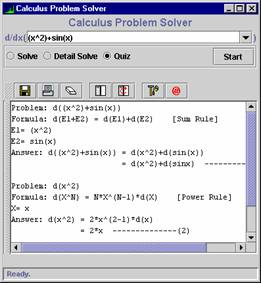Detailed step-by-step solution by using the above formulas;
• Interactive Quiz with multiple choice and fill-in-blank questions;
• Save Solutions in Text Format;
• Print Solutions;
• Multiplatform (works on any machine that can handle Java Virtual Machine);

 30-days Money Back Guarantee: We care about your satisfaction and for that all our products are subject to a 30-days money back guarantee. If for any reason you were not satisfied with a purchased product, we will refund you within 24 hours of receiving your refund request. For refund, email us at support@runiter.com with your name and order id.

See our other products:

Copyright (c) 2004 - Runiter Company - support@runiter.com